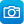# Mathematics / Year 8 / Measurement and Geometry / Geometric reasoning

Curriculum content descriptions

Define congruence of plane shapes using transformations (ACMMG200)

Elaborations
• understanding the properties that determine congruence of triangles and recognising which transformations create congruent figures
• establishing that two figures are congruent if one shape lies exactly on top of the other after one or more transformations (translation, reflection, rotation), and recognising that the matching sides and the matching angles are equal
General capabilities
• Literacy Literacy
• Numeracy Numeracy
ScOT terms

Congruence (Geometry)

## Refine results by

Year level 7-8
Resource type
Learning area Mathematics

## Refine by topic

Related topic### Congruent triangles

Find out about congruent and non-congruent triangles and the conditions required to make them. Use line segments and angles to build two congruent triangles for three different combinations of sides and angles. Explore the SSS case (side, side, side), the SAS case (two sides and the included angle) and the ASA case (two ...### Geometric reasoning - congruence

This is a website designed for both teachers and students that introduces congruence of shapes in the plane through transformations. In particular, transformations, translations, reflections in an axis and rotations of multiples of 90 degrees are used to define congruence and to identify congruent shapes. The four congruence ...### BBC Bitesize: transformations - revision

This is a set of illustrated information sheets with a focus on congruence and transformations. Examples with answers are included and students have access to multiple-choice questions to assess their learning. This resource is one of a series of online resources from the BBC's Bitesize collection.### The Mathematical Toolkit

A 2D Shapes tool that can be used to create geometric objects such as quadrilaterals, circles, triangles, lines, arcs, rays, segments and vectors on a coordinate grid. Plot and label the vertices to reveal the internal angles, side lengths, area and perimeter, then manipulate the shapes on a grid to transform their shape ...### TIMES Module 14: Measurement and Geometry: congruence - teacher guide

This is a 30-page guide for teachers. It contains an introduction to congruence, in particular the congruence tests for triangles. Applications of congruence are included throughout the module.### Planes of Symmetry

An animated tutorial demonstrating planes of symmetry of solids. An interactive quiz is included.### Symmetry and pattern: the art of oriental carpets

This resource is a website about the study of symmetry through analysing patterns in oriental carpets. It presents a gallery of different oriental rugs. Details about the patterns in each rug can be accessed by selecting the image of the rug or the key below the images. There are links to pages that explain symmetry and ...This series of six lessons explores geometry using real world contexts focussed on the dynamics of linkages and moving joints of everyday tools and objects. Students use physical models and computer simulations, the lessons move from a view of geometry as a study static diagrams to encompass movement. Each lesson is outlined ...### TIMES Module 21: Measurement and Geometry: rhombuses, kites and trapezia - teacher guide

This is a 32-page guide for teachers. It contains the definitions, properties and tests for rhombuses, kites and trapezia. Proofs of the properties and tests are given. Constructions for rhombuses, kites and trapezia are provided.### TIMES Module 22: Measurement and Geometry: scale drawings and similarity - teacher guide

This is a 41-page guide for teachers. It contains an introduction to scale drawings and similarity, and in particular the tests for triangles to be considered similar. Applications of similarity are included throughout the module.### Starting Smart: what are the properties of shapes and objects?

This curriculum resource package is a ten-week middle years teaching plan and set of supporting resources to extend and develop students' knowledge, understanding and application of circles, polygons and 3-D shapes. Students use interactive geometry software packages to define and construct circles and polygons from minimum ...